Courses

# Test: Forces & Laws of Motion- 4

## 25 Questions MCQ Test Science & Technology for UPSC CSE | Test: Forces & Laws of Motion- 4

Description
This mock test of Test: Forces & Laws of Motion- 4 for UPSC helps you for every UPSC entrance exam. This contains 25 Multiple Choice Questions for UPSC Test: Forces & Laws of Motion- 4 (mcq) to study with solutions a complete question bank. The solved questions answers in this Test: Forces & Laws of Motion- 4 quiz give you a good mix of easy questions and tough questions. UPSC students definitely take this Test: Forces & Laws of Motion- 4 exercise for a better result in the exam. You can find other Test: Forces & Laws of Motion- 4 extra questions, long questions & short questions for UPSC on EduRev as well by searching above.
QUESTION: 1

### Match the following with correct response.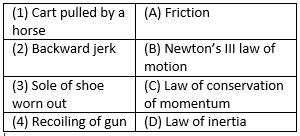Solution: 1) A cart is pulled by the horse as the horse applies a force on earth and earth applies a equal and opposite force. this is stated in Newton third law of motion. 2)We get a backward jerk due to inertia as the car speeds up and our body tends to move backwards and resist the change. 3)Sole of shoe worns out due to friction. 4)The spring in the gun transfers the momentum to the bulletand according to law of conservation of momentum the gun recoils.
QUESTION: 2

### Dust particles come out of a hanging carpet when we strike it with a stick because- A. Carpet starts moving forward and backward due to inertia of motion  B. Dust particles remain at rest  C. Dust particles starts moving forward due to inertia of motion D. Carpet remains at rest

Solution: This is because when we hit a carpet the carpet starts moving forward and its inertia of rest changes into the inertia of motion but the dust particles which are there with the carpet they move just because of the carpet but actually the particles remain at rest that is why these particles fall of due to the change of inertia.
QUESTION: 3

### Find the incorrect statement about Newton’s law of action and reaction. Action and reaction

Solution:

Newton’s third law of motion explains action and reaction. Action and reaction acts simultaneously, are equal in magnitude. They act on different direction and acts on different body.

QUESTION: 4

If an object experiences a net zero unbalanced force, than the body

Solution:

If an object experience a net zero unbalanced force, than the body moves with constant velocity. Zero unbalanced force due to produce acceleration in the body.

QUESTION: 5

Match the following with correct response.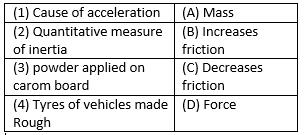Solution:

D is the correct option.Force is the cause of acceleration. Mass Is the quantitative measure Of inertia. We apply powder on the carrom board to decrease friction. Tyres of vehicles made rough to increase friction.

QUESTION: 6

A force of 7 kg-wt acts on a body of mass 10 kg calculate the acceleration produced.

Solution:

Force = 7 kg-wt = 7×10 = 70 N, mass = 10 kg. therefore, acceleration produced in the body = F/a = 70/10
= 7 ms-2.

QUESTION: 7

Which is incorrect statement about action and reaction referred to Newton’s third law of motion?
A. They are equal
B. They are opposite
C. They act on same object
D. They act simultaneously

Solution:

Newton’s third law states that for every action (force) in nature there is an equal and opposite reaction. In other words, if object A exerts a force on object B, then object B also exerts an equal and opposite force on object A.

For every action, there is an equal and opposite reaction. The statement means that in every interaction, there is a pair of forces acting on the two interacting objects. The size of the forces on the first object equals the size of the force on the second object.

The objects interact. The action and reaction force always act on different objects. Two forces acting on the same object, even if they have the same magnitude and point in opposite directions, never form an action-reaction pair.

QUESTION: 8

Statement A: A passenger falls forward when a bus suddenly starts moving in the forward direction
Statement B: A gun recoils backward with small speed than the bullet moving forward.

Solution:

A passenger falls backward when a bus suddenly start moving in the forward direction due inertia of rest. A gun recoils backward with small speed than the bullet moving forward due to law of conservation of momentum.

QUESTION: 9

When a force is exerted on an object, it can change its-
A. State
B. Position
C. Shape
D. Direction

Solution:

D is the correct option.A force acting on an object causes the object to change its shape or size, to start moving, to stop moving, to accelerate or decelerate. When there's the interaction between two objects they exert a force on each other, these exerted forces are equal in size but opposite in direction.

QUESTION: 10

The physical quantity which is equal to the rate of change of momentum is

Solution:

Force is the equal to the rate of change of momentum. Force = final momentum-initial momentum/time , f = mv-mu/t.

QUESTION: 11

Which of the following is not used to reduce friction

Solution:

To reduce the friction, oiling between contact surface, using ball bearing and using grease between contact surfaces. Making scratches between contact surfaces increase the friction.

QUESTION: 12

Match the following with correct response.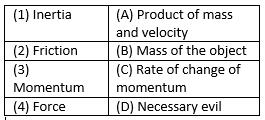Solution:
QUESTION: 13

The instruments that make our work easier are called

Solution:
QUESTION: 14

Which of the following physical quantity is associated with Newton’s second law of motion?

Solution:

Newton’s second law of motion states that rate of change in momentum is equal to force applied. Momentum is the product of mass and velocity. So, mass, velocity and time all are associated with Newton’s second law of motion.

QUESTION: 15

What is the momentum of an object of mass 1/2 m, moving with a velocity 2v?

Solution:

Momentum of a body is equal to product of mass and velocity. Momentum​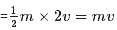QUESTION: 16

Match the following with correct response.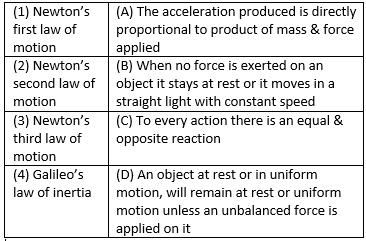Solution:
QUESTION: 17

An Object moving along a straight path with an accelerated motion. The correct statement about the object-
A. Its velocity keeps changing
B. Its speed keeps changing
C. A force is always acting on it
D. Its mass keeps on changing

Solution:

The correct option is Option C.
This is because when acceleration increases so will speed and velocity. As acceleration is a type of accelerated force a force will always act on the body.

QUESTION: 18

State whether the following statement is true or false “force can be measured using first law of motion”

Solution:

The force can be measured by newton’s second law of motion. First law of motion states that object in rest or uniform motion tends to remain in its state.

QUESTION: 19

“Internal forces are forces which bodies exert on each other when the bodies are part of the system” is

Solution:

Internal forces are those forces which exert on each other when the bodies are part of the system. The forces acting between the atoms of molecules that keep them together are example of internal force.

QUESTION: 20

Match the following with correct response.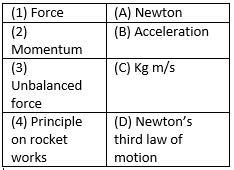Solution:
QUESTION: 21

The passenger feels a jerk forward when a bus moving at a high speed suddenly stops. This happens due to

Solution:

When a moving bus at high speed suddenly stops, the passenger feels jerk in forwarding direction due to the inertia of motion, which tends to keep the moving body in motion.

QUESTION: 22

“A body moves with a constant speed of 5m/s. This shows that no unbalanced forces are acting on the body” is a

Solution:

If no unbalance force acts on the body, the speed of body remain constant. So, a body moving with constant speed of 5m/s does not have any unbalanced force acting on it.

QUESTION: 23

Match the following with correct response.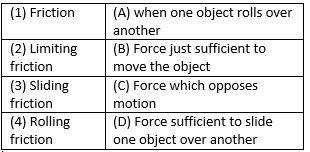Solution:
QUESTION: 24

Weight of a body due to the Earth acts

Solution:

The weight of the body is the measure of force by which object is attracted towards the centre of earth. It always acts vertically downwards.

QUESTION: 25

Same amount of force is acting on a car and bus. It the ratio of the mass of the bus and the car is 50:1, calculate the ratio of the acceleration produced in the car to the acceleration produced in the bus

Solution:

F1= mass of car x acceleration produced in the car =m1*a1
F2= mass of bus x acceleration produced in the bus =m2*a2
m2/m1= 1:50
since F1= F2
m1*a1 =m2*a2
a1/a2 =m2/m
=1:50